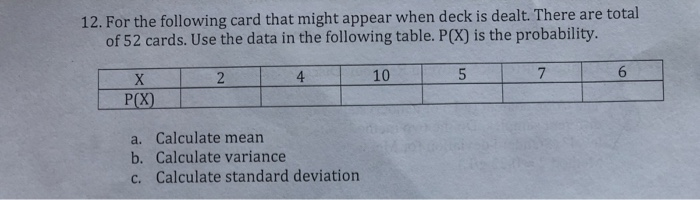# Question 12. For the following card that might appear when deck is dealt. There are total of 52 cards. Use the data in the following table. P(X) is the probability. 10 5 P(X) a. Calculate mean b. Calculate variance c. Calculate standard deviationOTEUAL The Asker · Probability and StatisticsTranscribed Image Text: 12. For the following card that might appear when deck is dealt. There are total of 52 cards. Use the data in the following table. P(X) is the probability. 10 5 P(X) a. Calculate mean b. Calculate variance c. Calculate standard deviation
More
Transcribed Image Text: 12. For the following card that might appear when deck is dealt. There are total of 52 cards. Use the data in the following table. P(X) is the probability. 10 5 P(X) a. Calculate mean b. Calculate variance c. Calculate standard deviation